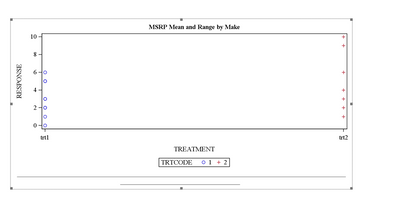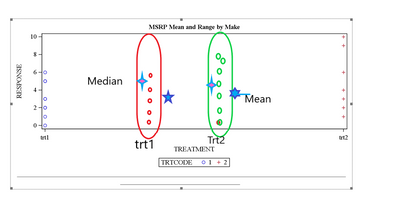## Scatter plot matrix axes

I have 5 variables with the same units that I'd like to create a scatterplot matrix for with regression lines. I used PROC SGSCATTER below. Is there a way to set the x- and y- axis ranges from 0 to 1 by 0.25?

``````data have ( keep = id var_: );
set sashelp.bweight;
if _N_ <= 20;
id = _N_;
var_a = ranuni ( 1 );
var_b = ranuni ( 2 );
var_c = ranuni ( 3 );
var_d = ranuni ( 4 );
var_e = ranuni ( 5 );
label var_a = "Variable A"
var_b = "Variable B"
var_c = "Variable C"
var_d = "Variable D"
var_e = "Variable E";
run;

proc sgscatter data = have;
compare y = ( var_a var_b var_c var_d var_e )
x = ( var_a var_b var_c var_d var_e ) /
reg spacing = 4;
run; quit;``````
1 ACCEPTED SOLUTION

Accepted Solutions

## Re: Scatter plot matrix axes

No, not explicitly, because the axes depend on the data. But if your data are always between [0,1] you can do the following:

1. Add "fake" data that won't be plotted but will force the axes to use [0,1] on each axis.

2. Make sure the scatter plot matrix is large enough that the horiz ticks do not get auto-thinned:

``````/* 1. Force [0,1] for each axis */
data Fake;
var_a = 0; var_b = .; var_c = .; var_d = .; var_e = .; output;
var_a = 1; var_b = .; var_c = .; var_d = .; var_e = .; output;
var_a = .; var_b = 0; var_c = .; var_d = .; var_e = .; output;
var_a = .; var_b = 1; var_c = .; var_d = .; var_e = .; output;
var_a = .; var_b = .; var_c = 0; var_d = .; var_e = .; output;
var_a = .; var_b = .; var_c = 1; var_d = .; var_e = .; output;
var_a = .; var_b = .; var_c = .; var_d = 0; var_e = .; output;
var_a = .; var_b = .; var_c = .; var_d = 1; var_e = .; output;
var_a = .; var_b = .; var_c = .; var_d = .; var_e = 0; output;
var_a = .; var_b = .; var_c = .; var_d = .; var_e = 0; output;
run;

data Want;
set Fake Have;
run;

/* 2. Prevent auto-thinning of tick marks */
ods graphics / width=1000px height=800px;
proc sgscatter data = Want;
compare y = ( var_a var_b var_c var_d var_e )
x = ( var_a var_b var_c var_d var_e ) /
reg spacing = 4 grid;
run;``````
8 REPLIES 8

## Re: Scatter plot matrix axes

No, not explicitly, because the axes depend on the data. But if your data are always between [0,1] you can do the following:

1. Add "fake" data that won't be plotted but will force the axes to use [0,1] on each axis.

2. Make sure the scatter plot matrix is large enough that the horiz ticks do not get auto-thinned:

``````/* 1. Force [0,1] for each axis */
data Fake;
var_a = 0; var_b = .; var_c = .; var_d = .; var_e = .; output;
var_a = 1; var_b = .; var_c = .; var_d = .; var_e = .; output;
var_a = .; var_b = 0; var_c = .; var_d = .; var_e = .; output;
var_a = .; var_b = 1; var_c = .; var_d = .; var_e = .; output;
var_a = .; var_b = .; var_c = 0; var_d = .; var_e = .; output;
var_a = .; var_b = .; var_c = 1; var_d = .; var_e = .; output;
var_a = .; var_b = .; var_c = .; var_d = 0; var_e = .; output;
var_a = .; var_b = .; var_c = .; var_d = 1; var_e = .; output;
var_a = .; var_b = .; var_c = .; var_d = .; var_e = 0; output;
var_a = .; var_b = .; var_c = .; var_d = .; var_e = 0; output;
run;

data Want;
set Fake Have;
run;

/* 2. Prevent auto-thinning of tick marks */
ods graphics / width=1000px height=800px;
proc sgscatter data = Want;
compare y = ( var_a var_b var_c var_d var_e )
x = ( var_a var_b var_c var_d var_e ) /
reg spacing = 4 grid;
run;``````

## Re: Scatter plot matrix axes

Cool trick, thank you!

## Re: Scatter plot matrix axes

The trick has many uses. For a discussion and other applications, see "A simple trick to include (and order!) all categories in SGPLOT legends."

## Re: Scatter plot matrix axes

Hi, I am creating a scatter plot.  I am new to the  different types of graphs.  When I run the code, I am able to generate the graph, however I am not sure how I can achieve that.

1. How I can move the treatments in to middle instead . Presently it printing at the both end of x axis.

2. Please ignore the circle made in green and red. I Just want to show where I want to display the new data points.

3. I need to display the Mean value right to the trt on x axis and median to the left to the trt ( Make sure these are not in the same x axis of data points or too far from the data points.. How I can achieve this because we did not given any values on X axis.

Fig 1 How it prints

fig2 How I want``````data test;
input Treatment\$ trtcode  response;
cards;
trt1 1 5
trt1 1 2
trt1 1 3
trt1 1 5
trt1 1 6
trt1 1 1
trt1 1 3
trt1 1 2
trt1 1 0
trt2 2 2
trt2 2 3
trt2 2 4
trt2 2 6
trt2 2 1
trt2 2 9
trt2 2 10
;
run;
ods graphics on/ width= 8in height= 4in   ;
options orientation = landscape errors = 2 missing = ' ' nofmterr ls = 175 validvarname = upcase nofmterr nobyline
noquotelenmax ;
ods results on;
ods listing close;
ods rtf file = "\&location.\dotted-plot.rtf";
proc sgplot data=test;
scatter x=treatment y=response / group=trtcode;
run;
ods _all_ close;``````

``````data test;
input Treatment\$ trtcode  response;
cards;
trt1 1 5
trt1 1 2
trt1 1 3
trt1 1 5
trt1 1 6
trt1 1 1
trt1 1 3
trt1 1 2
trt1 1 0
trt2 2 2
trt2 2 3
trt2 2 4
trt2 2 6
trt2 2 1
trt2 2 9
trt2 2 10
;
run;
ods graphics on/ width= 8in height= 4in   ;
options orientation = landscape errors = 2 missing = ' ' nofmterr ls = 175 validvarname = upcase nofmterr nobyline
noquotelenmax ;
ods results on;
ods listing close;
ods rtf file = "\&location.\dotted-plot.rtf";
proc sgplot data=test;
scatter x=treatment y=response / group=trtcode;
run;
ods _all_ close;``````

## Re: Scatter plot matrix axes

Compute the mean and median, then merge the statistics and the data. As for moving the graphics towards the center of the display, use the OFFSETMIN= and OFFSETMAX= options in the XAXIS statement:

``````/* compute the means and medians for each treatment group */
proc means data=test;
class Treatment;
var response;
output out=MeansOut mean=Mean median=Median;
run;

/* merge the data and the statistics */
data All;
set test MeansOut(where=(_Type_=1));
run;

/* use three data points: one for the data and one for each statistic */
proc sgplot data=All;
scatter x=treatment y=response;
scatter x=Treatment y=mean / markerattrs=(symbol=Plus size=16);
scatter x=Treatment y=median / markerattrs=(symbol=Star size=16);
xaxis type=discrete offsetmin=0.25 offsetmax=0.25;
run;``````

## Re: Scatter plot matrix axes

Hi @Rick_SAS . Thanks for  solution. I really appreciate it. However I want to do display the mean median on each side of the data points ( response) on x- axis, no same axis of the data points ( to avoid overlapping).

## Re: Scatter plot matrix axes

use the DISCRETEOFFSET= option

``````proc sgplot data=All;
scatter x=treatment y=response;
scatter x=Treatment y=mean / markerattrs=(symbol=Plus size=16) discreteoffset=-0.1 ;
scatter x=Treatment y=median / markerattrs=(symbol=Star size=16) discreteoffset=0.1;
xaxis type=discrete offsetmin=0.25 offsetmax=0.25;
run;
``````

## Re: Scatter plot matrix axes

Perfect. Thank you very much.
Discussion stats
• 8 replies
• 558 views
• 3 likes
• 3 in conversation